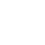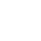# Math Chat

Zeros, Ping-Pong Balls, And Acceleration

Old challenge

5! = 5x4x3x2x1 = 120, which ends in a zero. Determine the number of zeros at the end of 25! (What about 510!)

25! ends in 6 zeros and 510! ends in 2,441,406 zeros. To understand why, start with the easier case of 10! = 10x9x8x7x6x5x4x3x2x1 = 3,628,800. It ends in two zeros, one because of the factor of 10 and one because of the separate factors 5x2. Since twos are much more common than fives, it is the number of factors of five that determines the number of terminal zeros. In 25!, 25/5 = 5 of the factors are divisible by 5, and one (namely 25) is divisible by 5 twice, for a total of 6 terminal zeros.

In 510!, 510/5 = 59 factors are divisible by 5, 510/52 = 58 are divisible by 5 twice, 510/53 = 57 are divisible by 5 three times, and so on, for a total T of T = 59 + 58 + 57 + ... + 51 + 1.

To compute T easily, note that 5T = 510 + 59 + 58 + ... + 51. When you subtract T from 5T, most terms cancel, and you get 4T = 510 - 1 and T = (510 - 1)/4 = 2,441,406. In general 5N! has (5N - 1)/4 terminal zeros. (D. Berquist, E. Brahinsky, J. Heine, J. Morrison, E. Randolph, J. Smit, D. Soskis, and M. Tiscareno all had the correct answer.)

More on ping-pong balls

B. Perot writes, "Regarding the ping-pong balls [Aug. 8] ... It's not enough to say that eventually every ball could be removed (that is certainly correct), but only part of the equation. In the limit as every ball is removed - it is also replaced by two others."

Math Chat replies: If you rightly agree that in one scenario every ball is eventually removed (and replaced by two later ones), and you think that at the end infinitely many remain, I challenge you to name one ball that remains. Ball 1 is removed at the first step, ball 2 is removed at the second step, ball 3 at the third step, and so on. Every ball is removed. No ball remains forever.

New challenge (M. Abel)

You are out driving your gas-powered or perhaps new electric battery-powered car. At a freeway entrance, you need to accelerate from 0 to 60 m.p.h. within 20 seconds. What do you think is the best way to do this to use the least amount of energy?

Math Chat

Fine Hall

Princeton, NJ 08540

or by e-mail to:

Frank.Morgan@williams.edu

You've read  of  free articles. Subscribe to continue.https://www.csmonitor.com/1997/0905/090597.feat.scitech.1.htmlhttps://www.csmonitor.com/subscribe

## Subscription expired

Your subscription to The Christian Science Monitor has expired. You can renew your subscription or continue to use the site without a subscription.

This message will appear once per week unless you renew or log out.

## Session expired

Your session to The Christian Science Monitor has expired. We logged you out.